Clutch Prep is now a part of Pearson
Ch.16 - Aqueous Equilibrium WorksheetSee all chapters

# Buffer

See all sections
Sections
Buffer
Acid and Base Titration Curves
Weak Acid Strong Base Titrations
Weak Base Strong Acid Titrations
Strong Acid Strong Base Titrations
Titrations of Diprotic and Polyprotic Acids
Ksp
Henderson Hasselbalch Equation (IGNORE)
###### Identifying a Buffer

A buffer is a solution composed of a weak acid with its conjugate base.

Concept #1: Understanding a Buffer.

Transcript

Hey guys! In this new video, we're going to take our first looks at what exactly is a buffer and how exactly do they work.
So we're going to say here, solutions that contain a weak acid and its conjugate base. And remember, when I say weak acid we could think of HF. Conjugate base means it has 1 less H+, so take off the H+ you have F-. But professors don't like to give you just F-, they'll combine that F- with a metal, so we could say our conjugate base here is NaF. So solutions which contain a weak acid like HF and a conjugate base has one less H like NaF are called buffer solutions because they resist drastic changes in pH.
Now knowing this, I want you guys to try to answer this first Practice Question. We're going to say: Which one of the following combinations does not create a buffer?
Remember we just set up above, a buffer is a weak acid and its conjugate base. So this is going to require you guys to remember the rules that we talked about in identifying compounds that is either being acidic or basic, strong or weak. Once you can do that, you will be able to guide your way to the correct answer for this one. Come back, click on the explanation button and see the choice that I make, and see if it matches up with yours.

Buffers resist drastic changes to the pH if a strong acid or strong base is added.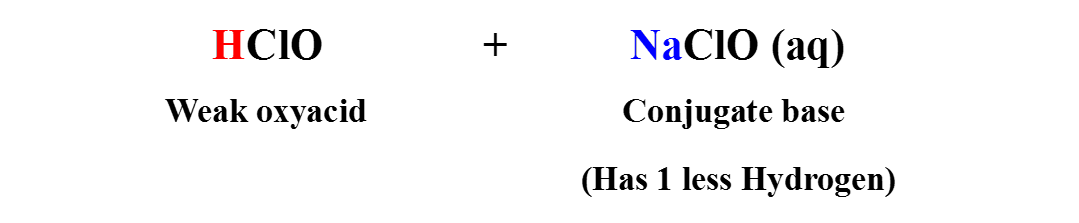If a strong base is added then the buffer resists a pH change by having the weak acid neutralize it.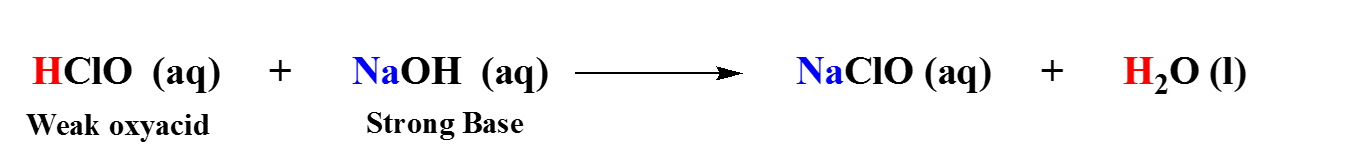If a strong acid is added then the buffer resists a pH change by having the conjugate base neutralize it.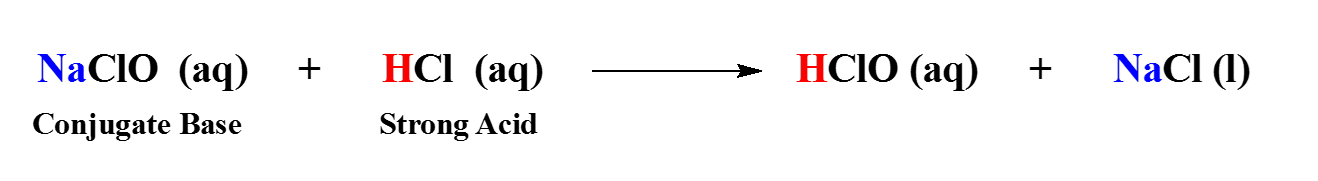Practice: Which one of the following combinations does not create a buffer?

Practice: Which of the following combinations can result in the formation of a buffer?

###### Buffer Creation

As we stated earlier a buffer is composed of a weak acid and its conjugate base, but there are actually three ways to create a buffer.

Concept #2: Method 1 for Creating a Buffer.

Transcript

Hey guys! In this new video, we're going to take a look at one of the different ways to create a buffer.
We know what a buffer is, a buffer is made up of a weak acid and conjugate base or a conjugate acid and a weak base, so that's what a buffer is. But there are 3 ways to make a buffer and it's going to be important that you guys recognize these 3 ways.
Now we're going to say the First Way is obvious: A buffer is made up of a weak acid and its conjugate base, so one way to make a buffer is just mixing those two things together.
So we're going to say mixing a weak acid and its conjugate base. So for Example:
What we need to realize here is that we're going to say in this case a good buffer, an ideal buffer, so ideal buffer means that this is the best type of buffer. An ideal buffer is when our weak acid = our conjugate base, they're the same amount. So let's say we have 0.1 M HF and 0.1 M NaF. And we should realize that the numbers could be different for them but they're work best when they're the same. We're going to say this has to do with our buffer range. What you should realize here is a good buffer has to fall within the range of 10:1 or 1:10. Okay, so what do I mean by that? I mean this.. So 10:1 that means our weak acid utmost could only be 10 times more than my conjugate base, if it falls outside that range it will be a bad buffer. So good buffer is between 10:1 ratio or 1:10 ratio. In the 1:10, now the weak acid is 1 and the conjugate base is 10 times that. Okay, so again this is the buffer range, a good buffer falls within this range. If it falls outside that range it'll still be a buffer, it will just be a very bad buffer. And remember, an ideal buffer is the best buffer, that's when both are the same.
Now connected to this also was another topic which is called our buffer capacity. Now we're going to say buffer capacity, all it means is the more concentrated my weak acid and conjugate base, the better my buffer. And this makes sense because remember what is the buffer doing? It's trying to neutralize the strong acid and the strong base that you add to them. And they can't do their job effectively if there's a little bit of them. So to fight such strong acids and strong bases, you need a lot of weak acid and a lot of conjugate base. The more the better, the more they can better defend themselves. So here we're going to say this is good because they're both the same number but this is better because it's more concentrated. So this has a better buffer capacity. So buffer capacity the more concentrated, the better.
Now if we go back to this ideal buffer, we're going to say that's when they both equal each other. We're going to say that this is found at our half-equivalence point. When we reach the half-equivalence point, that's when we have an ideal buffer. We'll talk more on detail on that when we get to titration graphs. When we get to the titration graphs of buffers, we'll take a close look and see what do I mean by the half-equivalence point and why is this an ideal buffer. So just for right now, we'll write a little note on that. An ideal buffer is when they're both equal, this happens at the half-equivalence point. So remember the difference between buffer capacity and buffer range. The best buffer is when they're both equal in amount. The buffer range says they could be a 10:1. Once it's outside that 10:1 ratio, the buffer is still a buffer but it's going to be a bad buffer. It'll get destroyed very quickly by a strong acid or base that we add.

The first and most obvious way to create a buffer is to simply combine a weak acid and its conjugate base. In this case, a buffer is most ideal when both components are highly concentrated and equal to one another.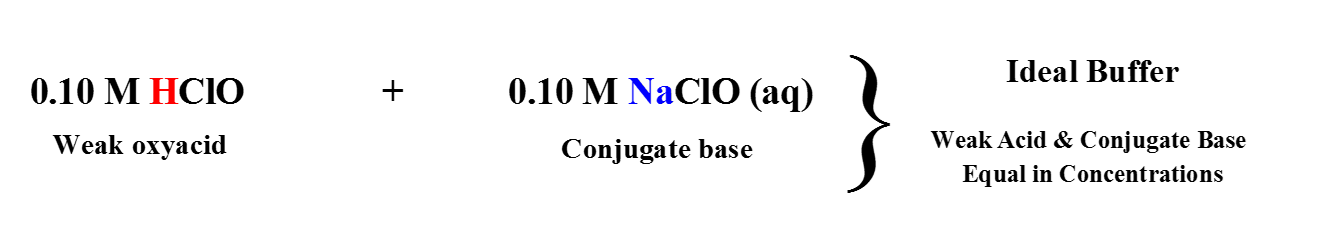The weak acid and conjugate base can be different from one another by up to a magnitude of 10. This is called the buffer range. If they are different by more than 10 then this is considered a bad buffer.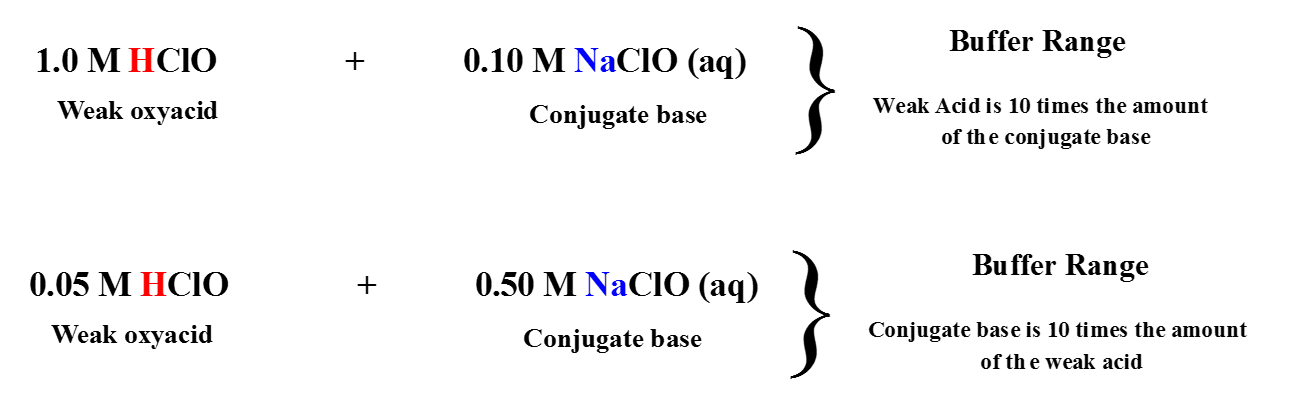Concept #3: Method 2 for Creating a Buffer.

Transcript

Now the Second Way to make a buffer is to mix some strong acid with some weak base. But here's the thing about this type, we're going to say here the weak base has to be higher in amount, must be higher in amount. If the weak base is equal to the strong acid, it'll destroy the buffer. If the strong acid is greater than my weak base, it'll destroy the buffer. So the weak base has to always be higher than the strong acid, otherwise the buffer will be destroyed.
So if we take a look at the Example we can say here:
We have 1 mol of HF and 1.5 mols of NH3. NH3 are weak base and there's more of it. Our units here can be mols or Molarity. In this case this would be a buffer. If we have 1 mol HF and 1 mol NH3, no longer a buffer because the strong species is equal to my weak species. If I have.. Sorry this is HCl actually. HF is a weak acid so disregard that, HCl. And if I have 1.5 mols of HCl and still 1 mol NH3, this will also no longer be a buffer. Your strong species cannot be equal to or greater than your weak species, if it is your buffer gets destroyed, so remember that. The weak species has to be higher in amount than the strong species.

The second method in creating a buffer is mixing a strong acid with a weak base. In this case since we have a strong species mixing with a weak species then we must make sure the weak species is higher in amount.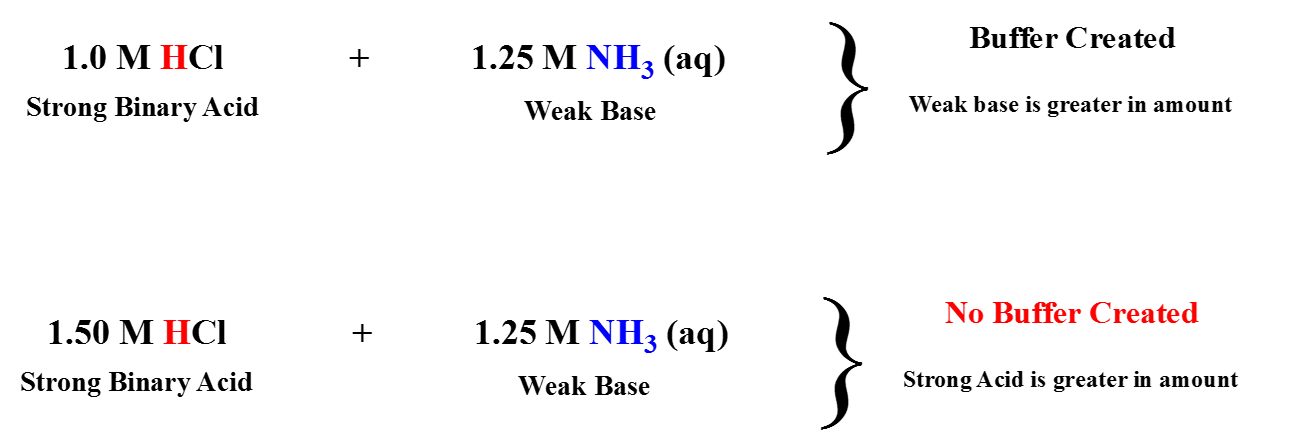Concept #4: Method 3 for Creating a Buffer.

Transcript

And then finally the last type, we just said strong acid and weak base. So, let's reverse it, weak acid and strong base. So example here, we could have 1.2, different number, 2.5 0 molar HF now, that's a weak acid and 1.0 molar NaOH, okay? So, now our weak acid is definitely larger in amount. So again, here we need the weak species higher in amount. So, this would be a buffer, got to remember that. So, if we go back first way is to mix weak acid and conjugate base here, they could be equal to or differ from each other but the good buffers are within the range of 10 to one, in the next two the weak species has to be higher than the strong species otherwise it won't be a buffer, you have to remember all three cases because you'll be tested on them and if you don't know which is which you won't be able to get the correct answer. So, just remember, weak acid conjugate base that can be equal that can be different as long as it's within the range of 10 to one it's a good buffer, if it's outside that range it's a bad buffer, case two and three the weak species have to be higher than the strong species, the strong species cannot be equal to or greater, if it is the buffer gets destroyed. Remember these principles and they'll help guide you to see if you have a buffer or not.

The third method in creating a buffer is mixing a strong base with a weak acid. In this case since we have a strong species mixing with a weak species then we must make sure the weak species is higher in amount.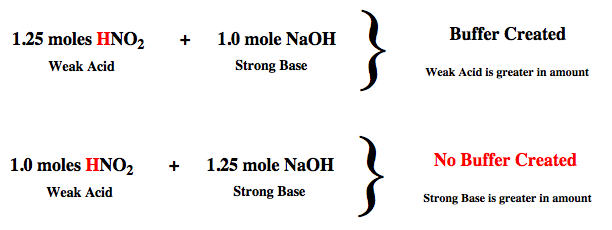Example #1: Which of the following combinations can result in the formation of a buffer?

a)     0.01 moles HClO (hypochlorous acid) and 0.05 moles of NaOH.

b)     0.01 moles HClO (hypochlorous acid) and 0.05 moles of HCl.

c)     0.01 moles HClO (hypochlorous acid) and 0.05 moles of NH3.

d)     0.01 moles HClO (hypochlorous acid) and 0.001 moles of NaOH

Transcript

Hey guys! In this new video, we're going to put to practice some of the principles we learned in creating a buffer.
So for Example 1 it says: Which of the following combinations can result in the formation of a buffer?
So what we should realize here is in every example we have 0.01 moles of hypochlorus acid (HClO). Now HClO it's an acid, so we know it's some type of acid. It has Oxygen so it's an oxy-acid. And remember if we do the math, 1 Oxygen - 1 Hydrogen gives us 0 left over. So HClO is a weak acid. So every single one of these is a weak acid. And because of that you have to remember what 2 ways can we make a buffer if we have a weak acid? So remember the 2 ways is if we have a weak acid and its conjugate base, and the second way is if we have a weak acid and a strong base. But in the second case, the weak species has to be higher in amount.
So let's take a look at the what's mixing with. Here we have NaOH which is a strong base. Here we have HCl which is a strong binary acid. Here NH3 is a neutral amine, so it's a weak base. And NaOH again is a strong base. So we're looking for the weak acid to be paired up with the conjugate base or the strong base. So automatically these 2 are out because we can't pair up a weak acid with a strong acid or weak acid with a weak base, it has to be a conjugate base or a strong base. Now in the first one we actually have more moles of these strong species, so this would not be a buffer, this would actually destroy the buffer. But for the last one, we have more of the weak species than the strong species, so would be the last one that makes a buffer. So again, remember the 3 principles that we learned in creating a buffer. Apply them to this type of question.
Now for Practice 1:
I want you guys to figure out which one of these combinations will result in a buffer. Here remember the word "of" means multiply. So if you multiply these 2 numbers together you can have to find moles because remember moles = Liters * Molarity so divide each of these by 1000, multiply it by the Molarity to have moles.
You could also keep it in milliliters and multiply times the Molarity but then that will give you millimoles. So I don't know if you guys want to deal with millimoles, it's basically the same thing but you don't have to do it that way. So divide them by a 1000, the mL to get L, multiply times the Molarity to give you moles.
And remember the 3 combinations that can result in the formation of a buffer. So check for each one of these, look to see do you have a weak acid and conjugate base; do you have a weak acid and strong base; or do you have a strong acid and weak base. Look for these combinations, if you don't see them don't bother doing the math. So I recommend looking at each compound first and seeing if they match up with any of these combinations. If they do, look more deeply, look to see does it help to create a buffer. If it doesn't match one of these 3 combinations, don't even bother looking at it, don't do the math at all. And remember one key thing I'll give you guys a hint, you have to find out to make sure you have the correct concentration of your strong bases. What do we say about strong bases? The concentration I give you is depended on how many ions you have of which particular for ions. Doing that will help to get the correct answer.
Once you're done with this, come back and click on the explanation button and watch me explain how to best approach this problem. Good luck guys!

Practice:  Which of the following combinations can result in the formation of a buffer?

Practice: A buffer solution is comprised of 50.0 mL of a 0.100 M HC2H3O2 and 60.0 mL of a 0.100 M NaC2H3O2. Which of the following actions would completely destroy the buffer?

###### Henderson – Hasselbalch Equation

Concept #5: The Henderson – Hasselbalch Equation.

Transcript

Hey guys, in this new video, we’re finally get to see how do we calculate the pH of a buffer. We’re going to say we learned that whenever we had a weak acid or a base, we have to use our favorite friend, the ICE chart in order to find pH or pOH. The great thing is now that we're dealing with buffers, we no longer necessarily need to do an ICE chart. We're going to say anytime we know we have a buffer for sure, we can skip the Ice chart altogether and just use our Henderson – Hasselbalch equation. So, Henderson-Hasselbalch. It's also known as the buffer equation. It says that pH equals pKa plus the log of conjugate base over weak acid. When I say pKa, remember P just means negative log. pKa equals the negative log of Ka. Here when I’m talking about conjugate base or weak acid, the units here can be either in molarity or in moles. It all depends. If they give you only molarity, use molarity. If they give you volumes of a particular molarity, remember the word of means multiply. We’d multiply liters times molarity to give ourselves moles.

Whenever we have a buffer we can skip the ICE Chart and use the Henderson Hasselbalch.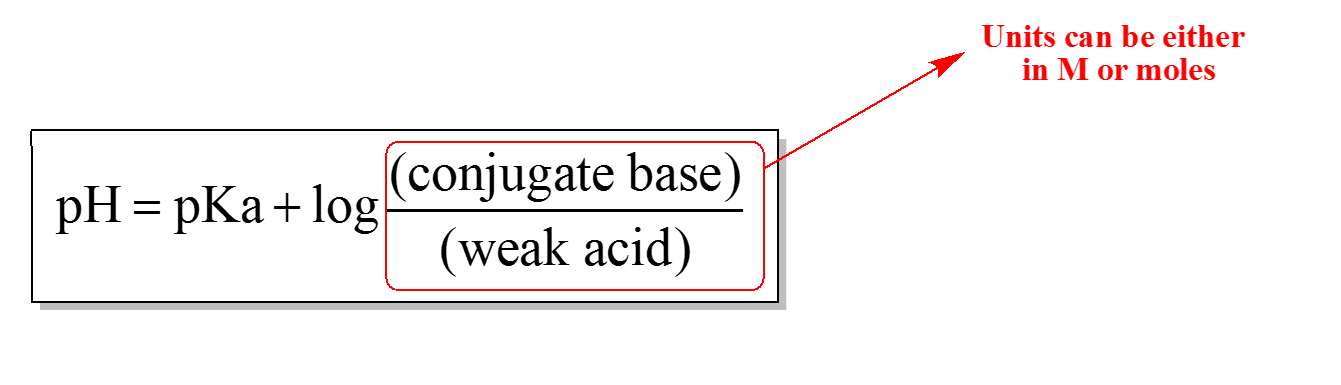Example #2: What is the pH of a solution consisting of 2.75 M sodium phenolate (C6H5ONa) and 3.0 M phenol (C6H5OH). The Ka of phenol is 1.0 x 10-10

Transcript

Let's look at this first question and see how exactly do we use it. Here it says: What is the pH of a solution consisting of 2.75 molar sodium phenolate, which is this compound, and 3.0 molar phenol, which is this compound. The Ka of phenol is 1.0 times 10 to the negative 10. What you should realize here is phenol has what? It has H's, non-metals, and oxygen. Phenol is an oxyacid. Use the Math that we've learned. You take the number of oxygens minus by the number of hydrogens. We don't get two oxygens left, so this is a weak oxyacid. We definitely know it’s weak because look at its Ka. Its Ka value is extremely small. If your Ka value is less than 1, you're a very weak acid.
Here we have a weak acid. sodium phenolate has one less H available, so this is the conjugate base. We have a weak acid, we have a conjugate base and therefore, we have a buffer. Because we have a buffer, we need to use the buffer equation, pH equals negative log of Ka plus the log of, now they only gave us the molarity here, so that's what we're going to plug in: 2.75 molar the conjugate base over 3.0 molar, our weak acid. When we plug all that in, we’ll get back the answer of 9.96

Practice: Calculate the pH of a solution formed by mixing 200 mL of a 0.400 M C2H5NH2 solution with 350 mL of a 0.450 M C2H5NH3solution. (Kb of C2H5NHis 5.6 x 10 -4).

Example #3: What is the buffer component concentration ratio, [Pr ] / [HPr] , of a buffer that has a pH of 5.11. (The Ka of HPr is 1.30 x 10-5).

Example #4: Over what pH range will an oxalic acid (H2C2O4) / sodium oxalate (NaHC2O4) solution work most effectively? The acid dissociation constant of oxalic acid is 6.0 x 10-2.

a) 0.22 – 2.22        b) 1.00 – 3.00        c) 0.22 – 1.22        d) 2.0 – 4.0

Practice: Determine how many grams of sodium acetate, NaCH3CO2 (MW: 82.05 g/mol), you would mix into enough 0.065 M acetic acid CH3CO2H (MW: 60.05 g/mol) to prepare 3.2 L of a buffer with a pH of 4.58. The Ka is 1.8 x 10-5.

Example #5: Which weak acid-conjugate base combination would be ideal to form a buffer with a pH of 4.74.

a)     Cyanic acid and Potassium cynate           (Ka = 4.9 x 10-10)

b)     Benzoic acid and Lithium benzoate         (Ka = 6.3 x 10-5)

c)     Acetic acid and Sodium acetate                   (Ka = 1.7 x 10-5)

d)     Ammonium chloride and Ammonia         (Ka = 5.56 x 10-10)

e)     Formic acid and Cesium formate                (Ka = 1.7 x 10-4)

Practice: A buffer solution is made by combining a weak acid with its conjugate salt. What will happen to the pH if the solution is diluted to one-fourth of its original concentration?